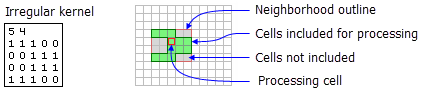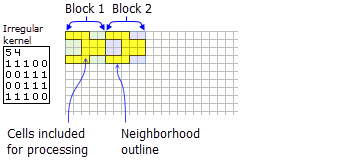# NbrIrregular

Mit der Spatial Analyst-Lizenz verfügbar.

## Zusammenfassung

Defines an irregular neighborhood which is created by a kernel file.

## AbbildungNbrIrregular neighborhood example for the FocalStatistics function.NbrIrregular neighborhood example for the BlockStatistics function.

## Auswertung

Tools that use neighborhood irregular object: Block Statistics and Focal Statistics.

##### Lizenz:

This class is also available if you have an Image Analyst extension license, but only for the Focal Statistics tool.

The kernel file specifies which cell positions should be included within the neighborhood.

For focal tools the x,y position for the processing cell within the neighborhood, with respect to the upper left corner of the neighborhood, is determined by the following equations:

`````` x = (width + 1)/2
y = (height + 1)/2``````

If the input number of cells is even, the x and y coordinates are computed using truncation.

The kernel file:

• The kernel file is an ASCII text file that defines the values and shape of an irregular neighborhood.

• The first line specifies the width and height of the neighborhood (the number of cells in the x-direction, followed by a space, and the number of cells in the y-direction).

• The subsequent lines give the values of each position in the neighborhood. The values are input in the same configuration as appears in the neighborhood they represent. A space between each value is necessary.

• The values in the kernel file should be either 0 or 1 . However, any value that is not 0 will be interpreted as 1.

• A value of 0 (not a blank space) for a cell position indicates that the cell is not a member of the neighborhood and will not be used for processing. A value of 1 indicates that its corresponding cell (and value) is a member of the neighborhood.

## Syntax

`NbrIrregular (inKernelFile)`
 Parameter Erklärung Datentyp inKernelFile The irregular inKernelFile is an ASCII text file that defines the shape of an irregular neighborhood. A value of 0 for a cell position indicates that the cell is not a member of the neighborhood, and a nonzero number at a corresponding cell's position indicates that the cell value be included as a member of the neighborhood. File

## Eigenschaften

 Eigenschaft Erklärung Datentyp inKernelFile(Lesen und schreiben) The irregular inKernelFile is an ASCII text file that defines the shape of an irregular neighborhood. A value of 0 for a cell position indicates that the cell is not a member of the neighborhood, and a nonzero number at a corresponding cell's position indicates that the cell value be included as a member of the neighborhood. String

## Codebeispiel

NbrIrregular example 1 (Python window)

Demonstrates how to create a NbrIrregular class and use it in the BlockStatistics tool within the Python window.

``````import arcpy
from arcpy import env
from arcpy.sa import *
env.workspace = "C:/sapyexamples/data"
outNbrKF = BlockStatistics("block", NbrIrregular("irreg.txt"))
outNbrKF.save("C:/sapyexamples/output/blstatsnbri2")``````
NbrIrregular example 2 (stand-alone script)

Implements the BlockStatistics tool using the NbrIrregular class.

``````# Name: NbrIrregular_Ex_02.py
# Description: Uses the NbrIrregular object to execute BlockStatistics tool.
# Requirements: Spatial Analyst Extension

# Import system modules
import arcpy
from arcpy import env
from arcpy.sa import *

# Set environment settings
env.workspace = "C:/sapyexamples/data"

# Set local variables
inRaster = "block"

# Create the Neighborhood Object
inKernelFile = "C:/data/irreg.txt"
myNbrIrreg = NbrIrregular(inKernelFile)

# Execute BlockStatistics
outBlkStat =  BlockStatistics(inRaster, myNbrIrreg, "MINIMUM", "DATA")

# Save the output
outBlkStat.save("C:/sapyexamples/output/blstat_irr3")``````